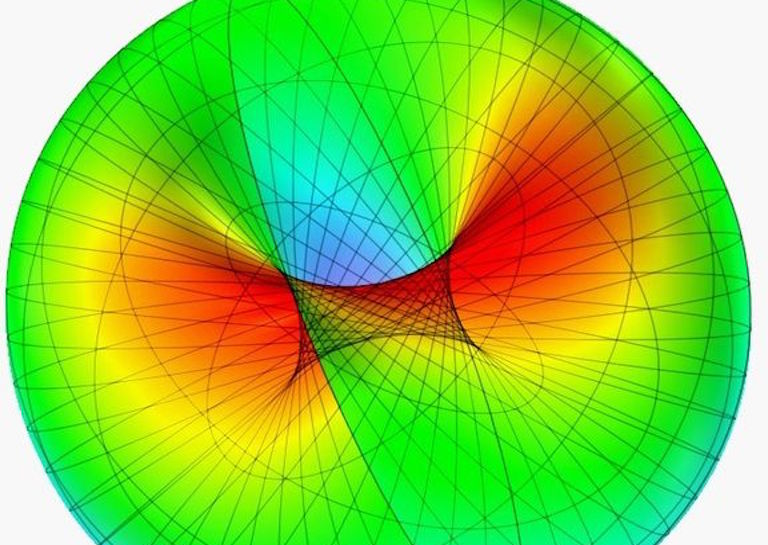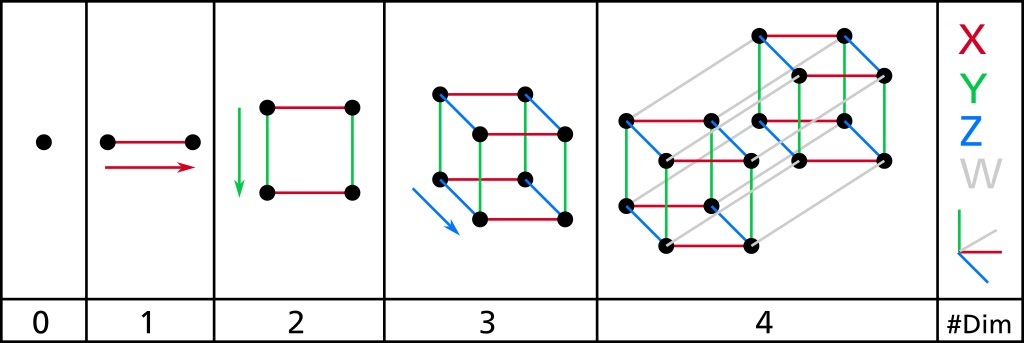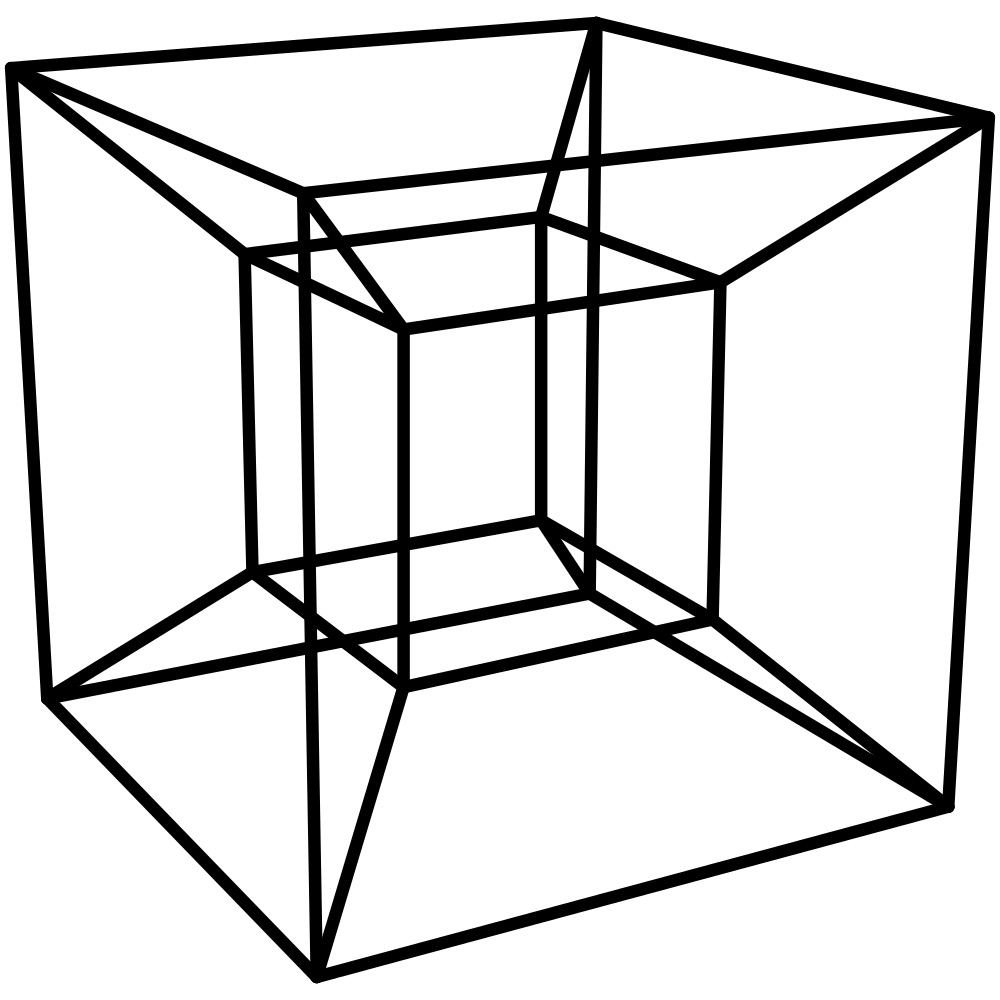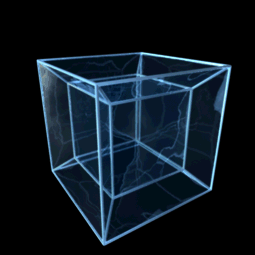# Understanding the Fourth Dimension From Our 3D Perspective

•The fourth dimension is a place you can travel to by going in a direction perpendicular to the third dimension. To the untrained eye, this statement makes absolutely no sense. How could there be a direction that is perpendicular to a three-dimensional space? In order to better understand this concept, we have to slowly work our way through all of the dimensions and analyze what changes are being made between each.[Image Source: Wikimedia]

## Zeroth Dimension

The zeroth dimension is one that we don't often think about. Points are the only dimensional beings that can be correctly understood in the zeroth dimension. They have absolutely no dimensions, no width, no length, no height. They are the smallest they could ever be, but also the largest they could ever be at the same time.

As we look to deepen our understanding of the fourth dimension. We can examine a cube in each dimension as we progress. A cube in the zeroth dimension would simply be a point. All of its dimensions are the same in every direction because there are none. The cube still represents a point in space, but that is the extent of its power in the zeroth dimension. Now, let's move into 1-D.

## First Dimension

The transition between the zeroth dimension and the first dimension involves an extrusion in any direction. In the first dimension, everything exists as a line. The only thing that differs between objects in the first dimension is their length. Lines all have the same width and the same height, but their length can be varied.

If you want to make lines varying thicknesses, then you have to move into 2-dimensional lines. A cube in the first dimension would look like a line with the same length as said cube, but no width or height values.

## Second Dimension

Transforming a line segment in a direction perpendicular to the 1-dimensional direction brings you into the second dimension. Keep this idea in mind as we expand our dimensional knowledge and notice this perpendicular action repeated as we move through dimensions.

In the second dimension, our cube can begin to look like a cube, but only just barely. A cube would exist as a square in the second dimension. You can, of course, draw a representation of a 3D cube in 2 dimensions, but that isn't what a cube would look like in 2 dimensions. Rather this would simply be a representation of the third dimension superimposed on the second.

Most Popular

Length and width can be varied in the second dimension, which allows for basic shapes and geometry. When we move into the third dimension, the math starts getting more complex.[Image Source: Wikimedia Commons]

## Third Dimension

The cube from the second dimension now gets extruded in a third perpendicular direction to both sides of the 2D square. To put this in cartesian terms, the 2D square existed in the X and Y directions. Moving into the 3rd dimension extruded that square in the Z direction. The third dimension is where our cube actually becomes a cube in our traditional defined sense. The object has dimensions of width, length, and height.

Throughout all of the dimensions, it is important to note that a cube will maintain all of its basic properties in theory. All of the angles will be right and all of the sides will be the same. Bringing in another principle of dimensions, we can examine what would occur if the cube was expanded indefinitely. When a cube in the third dimension is expanded to infinity, it encompasses the entirety of the 3-dimensional space.

So far, you should likely grasp these 3 dimensions, after all, they are the dimensions with which we most commonly associate.

## Fourth Dimension

When we bring the cube into the fourth dimension, we begin to experience some counterintuitive math. We extrude the cube in a direction perpendicular to all of the first three. This is impossible within the third dimension because there are only 3 dimensions which the cube is already expanded in. When we add the fourth dimension, in order to maintain the properties of the cube of all angles being 90 degrees and all sides being the same, we must extrude in this new dimension.

Cubes in the fourth dimensions are technically called tesseracts. Objects in 4D differ in length, width, height, and trength. Superimposing trength on any of the previous dimensions gives an object in the subsequent dimensions a trength of 0, or a value that is infinitely small.

All of the edges of a tesseract are the same, and all of the angles are right. This makes sense in theory, but when we begin to imagine what a tesseract would look like, we are bound by our 3-dimensional minds. To view a tesseract, we have to superimpose this fourth-dimensional object into the third dimension.

The main way we represent a tesseract, or fourth dimensional cube, is by projecting it into the third dimension with perspective. This representation can be seen below.[Image Source: Wikimedia]

This is also a perspective representation of a tesseract in gif form.[Image Source: Wikimedia]

This representation isn't what a 4D cube looks like, it is simply what one looks like in perspective viewed from the third dimension. To summarize our understanding of the fourth dimension, objects in 4D vary in value by length, width, height, and trength. All of these dimensional measures extend in a direction perpendicular to the previous three. Width is perpendicular to length, height is perpendicular to width and length, and finally, trength is perpedicular to height, length, and width.

These concepts are hard to grasp but hopefully, this gives you a good overview understanding of how the fourth dimension works and how we interpret it from our 3-dimensional eyes.

Still doesn't make any sense? Let Carl Sagan explain it to you.